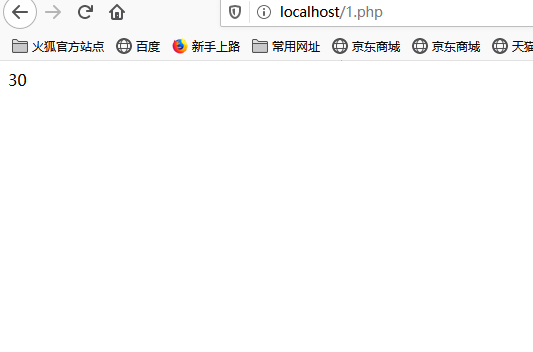# php运算符4，记住了哈，php7已经变量为输出为echo();了加,字符串为echo $a;了哈，记住啊啊啊 第一： 算术运算符中+ - * / 我们不用说了把，我们说点难的，说%还有++ --把，就说这几个 %无非这三种把，第一种是如果第一位大于第二位的话，就取余，你把它换算成10/3=3。。。。。。1，取余就是取后面的1啊 <?php$a=10%3;
echo $a; ?>  效果：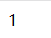第二种是如果第二位大于第一位的话，就直接取第一位的，为什么，因为除不了嘛是吧，所以取第一位的啊，因为都是第一位的换算得来的啊，算不了不就是取第一位的吗是吧 <?php$a=3%10;
echo $a; ?>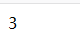//第三种，我问你一下，如果两种相同取什么，我说取0啊，为什么，因为1010=1…0啊，所以取0啊是吧 <?php$a=10%10;
echo $a; ?>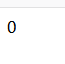然后说说++ – 我知道你们在困惑什么，我告诉你们++或者–在后面的运算把 a + + ; 的 原 理 是 什 么 呢 我 来 说 一 下 ， 最 简 单 的 解 释 哈 比 如 a++; 的原理是什么呢我来说一下，最简单的解释哈 比如 a初始值为2， $x=$a++;  我想问一下你们， a 多 少 ？ a多少？ x多少？ <?php$a=2;
$x=$a++;
echo '$x='.$x;
echo "<br>";
echo '$a='.$a;
?>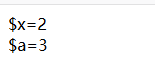<?php
$a=2;$x=$a--; echo '$x='.$x; echo "<br>"; echo '$a='.$a; ?>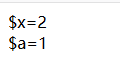运行原理：先赋值给 x , 然 后 是 x,然后是 a–幻化为 a = a= a-1;然后是2-1=1是吧，赋值给$a就行了啊，慢慢理解哈，兄弟们，慢慢就行了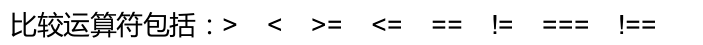<?php
var_dump(3=='3');
?>必须这样啊啊啊啊啊啊


<?php
var_dump(3==='3');
?>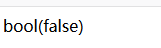var_dump(‘chengdu’ > ‘chongqing’);
var_dump(‘abcf’ > ‘abcd’);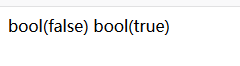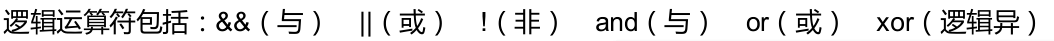&&:只要出现一个false就是false否则全部都是true就是true
||：出现两个true就是false，出现两个false就是false，出现一个false一个true就是true
!:true就是false，false就是true，相反的哈，往相反的来想哈
xor：意思是（一个）为true为真，(全真)或者(全假)为假哈

<?php
$a="Hello";$b="PHP";
$c=$a. $b; echo($c);
?>


<?php
$a="Hello";$b="PHP";
$c='aa'. 'hh'; echo$c;
?>


$b=array(4,5,6,7,8); var_dump($a+$b); ?>  记住哈，变量与变量值之间不能有空格哈！！！！！！！！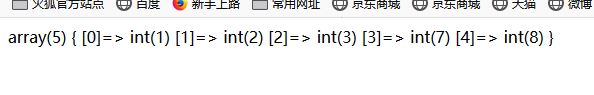屏蔽错误操作符 @： <?php$a=array(1,2,3);
echo(@$a); ?>  记住哈，php7已经有很大不同了记住了哈echo变量的哈加上echo();哈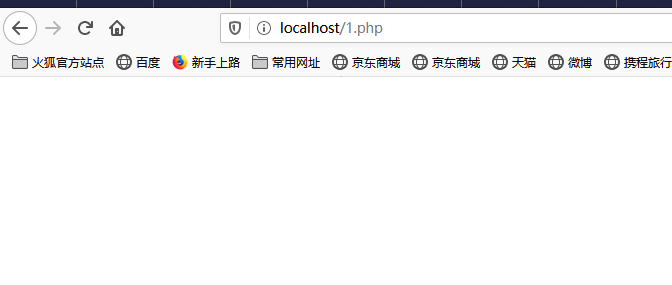赋值运算符: 赋值运算符包括：= .= += ­= *= *= /= %= &= |= ^= >>= <<= 其他就是右赋值给左边哈记住了哈！！！！！！ 也经常会使用到比如 =（乘等）、/=（除等）他们都是先先赋值然后算出本身的值哈，像$a++;一样哈，你看前面就知道了哈，这种垃圾我就不讲了

<?php
$a=5;$b=6;
$b*=$a;
echo(\$b);
?>